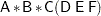Specification of Effects

In SAS analysis-of-variance procedures, the variables that identify levels of the classifications are called classification variables, and they are declared in the CLASS statement. Classification variables are also called categorical, qualitative, discrete, or nominal variables. The values of a classification variable are called levels. Classification variables can be either numeric or character. This is in contrast to the response (or dependent) variables, which are continuous. Response variables must be numeric.

The analysis-of-variance model specifies effects, which are combinations of classification variables used to explain the variability of the dependent variables in the following manner:

• Main effects are specified by writing the variables by themselves in the CLASS statement: A   B   C. Main effects used as independent variables test the hypothesis that the mean of the dependent variable is the same for each level of the factor in question, ignoring the other independent variables in the model.

• Crossed effects (interactions) are specified by joining the CLASS variables with asterisks in the MODEL statement: A*B   A*C   A*B*C. Interaction terms in a model test the hypothesis that the effect of a factor does not depend on the levels of the other factors in the interaction.

• Nested effects are specified by following a main effect or crossed effect with a CLASS variable or list of CLASS variables enclosed in parentheses in the MODEL statement. The main effect or crossed effect is nested within the effects listed in parentheses: B(A)   C*D(A B). Nested effects test hypotheses similar to interactions, but the levels of the nested variables are not the same for every combination within which they are nested.

The general form of an effect can be illustrated by using the CLASS variables A, B, C, D, E, and F:The crossed list should come first, followed by the nested list in parentheses. Note that no asterisks appear within the nested list or immediately before the left parenthesis.

Main Effects Models

For a three-factor main effects model with A, B, and C as the factors and Y as the dependent variable, the necessary statements are

proc anova;
class A B C;
model Y=A B C;
run;

Models with Crossed Factors

To specify interactions in a factorial model, join effects with asterisks as described previously. For example, these statements specify a complete factorial model, which includes all the interactions:

proc anova;
class A B C;
model Y=A B C A*B A*C B*C A*B*C;
run;

Bar Notation

You can shorten the specifications of a full factorial model by using bar notation. For example, the preceding statements can also be written

proc anova;
class A B C;
model Y=A|B|C;
run;

When the bar (|) is used, the expression on the right side of the equal sign is expanded from left to right by using the equivalents of rules 2–4 given in Searle (1971, p. 390). The variables on the right- and left-hand sides of the bar become effects, and the cross of them becomes an effect. Multiple bars are permitted. For instance, A | B | C is evaluated as follows:

 A | B | CA | B| CA  B  A*B| CA  B  A*B  C  A*C  B*C  A*B*C

You can also specify the maximum number of variables involved in any effect that results from bar evaluation by specifying that maximum number, preceded by an @ sign, at the end of the bar effect. For example, the specification A | B | C@2 results in only those effects that contain two or fewer variables; in this case, A  B  A*B  C  A*C and B*C.

The following table gives more examples of using the bar and at operators.

 A | C(B) is equivalent to A  C(B)  A*C(B) A(B) | C(B) is equivalent to A(B)  C(B)  A*C(B) A(B) | B(D E) is equivalent to A(B)  B(D  E) A | B(A) | C is equivalent to A B(A) C A*C  B*C(A) A | B(A) | C@2 is equivalent to A  B(A)  C  A*C A | B | C | D@2 is equivalent to A  B  A*B  C  A*C   B*C  D  A*D   B*D  C*D

Consult the section Specification of Effects in Chapter 41, The GLM Procedure, for further details on bar notation.

Nested Models

Write the effect that is nested within another effect first, followed by the other effect in parentheses. For example, if A and B are main effects and C is nested within A and B (that is, the levels of C that are observed are not the same for each combination of A and B), the statements for PROC ANOVA are

proc anova;
class A B C;
model y=A B C(A B);
run;

The identity of a level is viewed within the context of the level of the containing effects. For example, if City is nested within State, then the identity of City is viewed within the context of State.

The distinguishing feature of a nested specification is that nested effects never appear as main effects. Another way of viewing nested effects is that they are effects that pool the main effect with the interaction of the nesting variable.

See the "Automatic Pooling" section, which follows.

Models Involving Nested, Crossed, and Main Effects

Asterisks and parentheses can be combined in the MODEL statement for models involving nested and crossed effects:

proc anova;
class A B C;
model Y=A B(A) C(A) B*C(A);
run;

Automatic Pooling

In line with the general philosophy of the GLM procedure, there is no difference between the statements

model Y=A B(A);

and

model Y=A A*B;

The effect B becomes a nested effect by virtue of the fact that it does not occur as a main effect. If B is not written as a main effect in addition to participating in A*B, then the sum of squares that is associated with B is pooled into A*B.

This feature allows the automatic pooling of sums of squares. If an effect is omitted from the model, it is automatically pooled with all the higher-level effects containing the CLASS variables in the omitted effect (or within-error). This feature is most useful in split-plot designs.